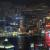# TypeScript 4.0 Beta

TypeScript 4.0 Beta 已发布，整体看来，此版本在兼容性方面没有特别大的变化。因为 TypeScript 团队表示新版本继续使用与过去版本相似的版本控制模型，可将 4.0 视作 3.9 的延续升级版本。而且他们也一直在追求不牺牲主要灵活性的情况下，提供一个最大限度减少 breaking changes 的升级路径。

``npm install typescript@beta``

4.0 主要新功能

``````class Square {
// Previously: implicit any!
// Now: inferred to `number`!
area;
sideLength;

constructor(sideLength: number) {
this.sideLength = sideLength;
this.area = sideLength ** 2;
}
}``````

``````class Square {
sideLength;

constructor(sideLength: number) {
if (Math.random()) {
this.sideLength = sideLength;
}
}

get area() {
return this.sideLength ** 2;
//     ~~~~~~~~~~~~~~~
// error! Object is possibly 'undefined'.
}
}``````

``````class Square {
// definite assignment assertion
//        v
sideLength!: number;
//         ^^^^^^^^
// type annotation

constructor(sideLength: number) {
this.initialize(sideLength)
}

initialize(sideLength: number) {
this.sideLength = sideLength;
}

get area() {
return this.sideLength ** 2;
}
}``````

JavaScript 和其他很多语言都支持复合赋值运算符。复合赋值运算符将一个运算符应用到两个参数上，然后将结果赋值到左边。如下：

``````/ Addition
// a = a + b
a += b;

// Subtraction
// a = a - b
a -= b;

// Multiplication
// a = a * b
a *= b;

// Division
// a = a / b
a /= b;

// Exponentiation
// a = a ** b
a **= b;

// Left Bit Shift
// a = a << b
a <<= b;``````

JavaScript 中的许多运算符都有一个对应的赋值运算符，但有三个例外：逻辑和(`&&`)、逻辑或(`||`)，以及空值合并(`??`)。

TypeScript 4.0 为上述三个运算符增加了对应的赋值运算符支持：

``````let values: string[];

// Before
(values ?? (values = [])).push("hello");

// After
(values ??= []).push("hello");``````
``````a ||= b;

// actually equivalent to

a || (a = b);``````

### 精彩评论u

D
ts牛逼

### 最新评论（7）u

D
ts牛逼